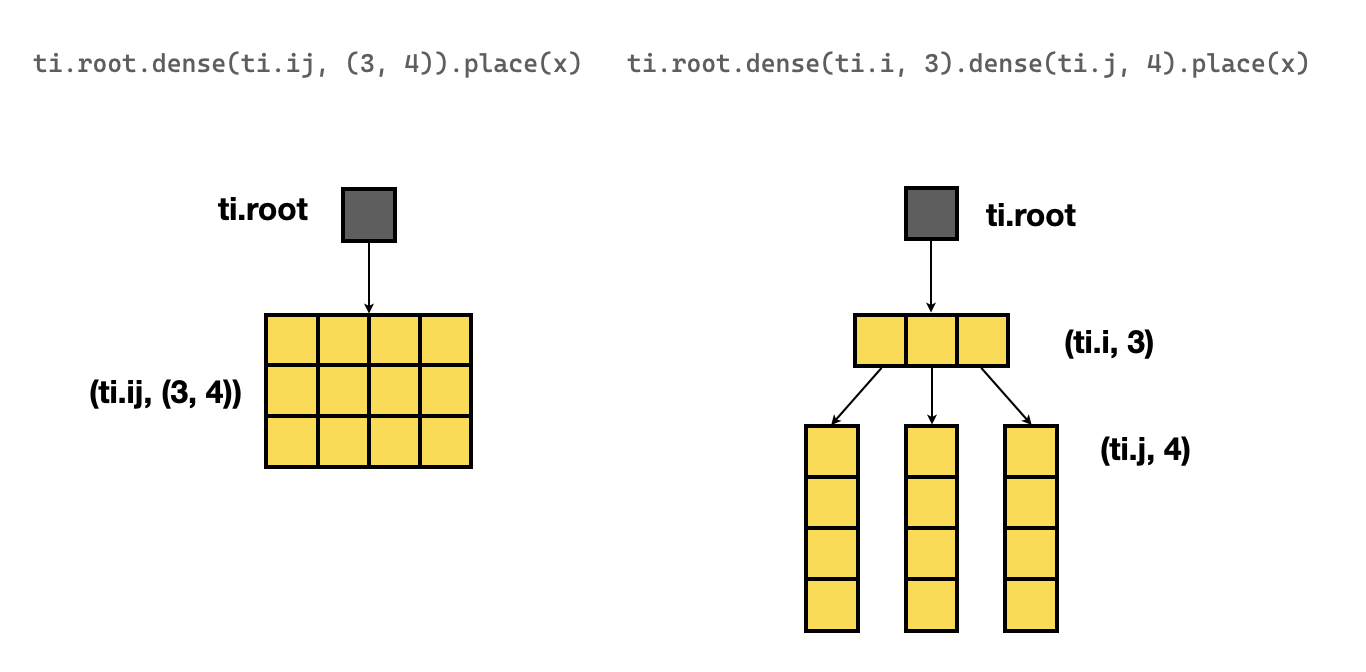Version: v1.6.0

# Field（进阶版）

## 组织高效的数据布局

• 稠密数据结构
• 小范围数据循环（对大多数处理器而言 32 KB 内）
• 顺序加载与存储数据

### 布局 101：从 `shape` 到 `ti.root.X`

• 声明一个零维 filed：
``x = ti.field(ti.f32)ti.root.place(x)# is equivalent to:x = ti.field(ti.f32, shape=())``
• 声明一个形状为 `3` 的一维 field：
``x = ti.field(ti.f32)ti.root.dense(ti.i, 3).place(x)# is equivalent to:x = ti.field(ti.f32, shape=3)``
• 声明一个形状为 `(3, 4)` 的二维 field：
``x = ti.field(ti.f32)ti.root.dense(ti.ij, (3, 4)).place(x)# is equivalent to:x = ti.field(ti.f32, shape=(3, 4))``

``x = ti.field(ti.f32)ti.root.dense(ti.i, 3).dense(ti.j, 4).place(x)# has the same shape withx = ti.field(ti.f32, shape=(3,4))``
##### note

``x = ti.field(ti.f32)ti.root.dense(ti.i, 3).dense(ti.j, 4).place(x)``

``x = ti.field(ti.f32)ti.root.dense(ti.ij, (3, 4)).place(x)# or equivalentlyx = ti.field(ti.f32, shape=(3,4))````for i, j in A:    A[i, j] += 1``

Taichi 编译器能够自动推断底层的数据布局并应用合适的数据读取顺序。 这是 Taichi 编程语言相较其他大多数通用编程语言的一大优势。在这些语言中，数据读取顺序只能手动优化。

### 行主序 vs. 列主序

Taichi field 的默认布局是行主序的。 你可以通过 `ti.root` 语句这样定义 field：

``x = ti.field(ti.f32)y = ti.field(ti.f32)ti.root.dense(ti.i, M).dense(ti.j, N).place(x)   # row-majorti.root.dense(ti.j, N).dense(ti.i, M).place(y)   # column-major``

``# address:  low ........................................... high#       x:  x[0, 0]  x[0, 1]  x[1, 0]  x[1, 1]  x[2, 0]  x[2, 1]#       y:  y[0, 0]  y[1, 0]  y[2, 0]  y[0, 1]  y[1, 1]  y[2, 1]``

##### note

``int x;  // row-majorint y;  // column-majorfor (int i = 0; i < 3; i++) {    for (int j = 0; j < 2; j++) {        do_something(x[i][j]);        do_something(y[j][i]);    }}``

`x``y` 的访问器在行主序数组和列主序数组之间分别为倒序。 与 Taichi field 相比，当你更改内存布局时，需要修改更多代码。

### AoS vs. SoA

AoS 全称 array of structures（数组结构体），SoA 全称 structure of arrays（结构体数组）。 一个带有四个像素和三个颜色通道的 RGB 图像：AoS 布局存储 `RGBRGBRGBRGB`，而 SoA 布局存储 `RRRRGGGGBBBB`。 选择 AoS 还是 SoA 布局很大程度上取决于 field 的访问模式。 让我们讨论一个处理大规格 RGB 图像的场景。 这两个布局的内存安排如下：

``# address: low ...................... high# AoS:     RGBRGBRGBRGBRGBRGB.............# SoA:     RRRRR...RGGGGGGG...GBBBBBBB...B``

``x = ti.field(ti.f32)y = ti.field(ti.f32)ti.root.dense(ti.i, M).place(x)ti.root.dense(ti.i, M).place(y)``

``#  address: low ................................. high#           x  x  x ... y  y  y ...``

``x = ti.field(ti.f32)y = ti.field(ti.f32)ti.root.dense(ti.i, M).place(x, y)``

``#  address: low .............................. high#           x  y  x  y  x  y ...``

``N = 200000pos = ti.field(ti.f32)vel = ti.field(ti.f32)# SoA placementti.root.dense(ti.i, N).place(pos)ti.root.dense(ti.i, N).place(vel)@ti.kerneldef step():    pos[i] += vel[i] * dt    vel[i] += -k * pos[i] * dt``

``N = 200000pos = ti.field(ti.f32)vel = ti.field(ti.f32)# AoS placementti.root.dense(ti.i, N).place(pos, vel)@ti.kerneldef step():    pos[i] += vel[i] * dt    vel[i] += -k * pos[i] * dt``

# SoA version

N = 200000 pos = ti.field(ti.f32) vel = ti.field(ti.f32) ti.root.dense(ti.i, N).place(pos) ti.root.dense(ti.i, N).place(vel)

@ti.kernel def step(): pos[i] += vel[i] dt vel[i] += -k pos[i] * dt

`````python# AoS versionN = 200000pos = ti.field(ti.f32)vel = ti.field(ti.f32)ti.root.dense(ti.i, N).place(pos)ti.root.dense(ti.i, N).place(vel)@ti.kerneldef step():    pos[i] += vel[i] * dt    vel[i] += -k * pos[i] * dt``

Here, `pos` and `vel` for SoA are placed separately, so the distance in address space between `pos[i]` and `vel[i]` is `200000`. This results in poor spatial locality and poor performance. A better way is to place them together:

``ti.root.dense(ti.i, N).place(pos, vel)``

Then `vel[i]` is placed right next to `pos[i]`, which can increase spatial locality and therefore improve performance. --><!-- For example, the following places two 1D fields of shape `3` together, which is called Array of Structures (AoS):

``ti.root.dense(ti.i, 3).place(x, y)``

Their memory layout is:

By contrast, the following places these two fields separately, which is called Structure of Arrays (SoA): --><!-- ```python ti.root.dense(ti.i, 3).place(x) ti.root.dense(ti.i, 3).place(y)

``Now their memory layout is:**To improve spatial locality of memory accesses, it may be helpful toplace data elements that are often accessed together withinrelatively close addresses.**  -->### AoS 扩展：层级结构的 field<!-- haidong: I hope to remove this subsection. This content just repeats the AoS topic --> 有时我们想要以某种复杂但固定的模式访问内存，比如在 8x8 的块中遍历图像。 表面上最佳做法是平展每个 8x8 的块并将它们连接在一起。 然而，此时 field 已不再是一个扁平缓存器，因为它有两个层级：图像层和块层。 也就是说，这个 field 是一个隐式 8x8 块结构的数组。我们用如下语句表示：```python# Flat fieldval = ti.field(ti.f32)ti.root.dense(ti.ij, (M, N)).place(val)``
``# Hierarchical fieldval = ti.field(ti.f32)ti.root.dense(ti.ij, (M // 8, N // 8)).dense(ti.ij, (8, 8)).place(val)``

## 管理内存占用

### Field 的手动分配和销毁

``import taichi as titi.init()@ti.kerneldef func(v: ti.template()):    for I in ti.grouped(v):        v[I] += 1fb1 = ti.FieldsBuilder()x = ti.field(dtype=ti.f32)fb1.dense(ti.ij, (5, 5)).place(x)fb1_snode_tree = fb1.finalize()  # Finalizes the FieldsBuilder and returns a SNodeTreefunc(x)fb1_snode_tree.destroy()  # Destructionfb2 = ti.FieldsBuilder()y = ti.field(dtype=ti.f32)fb2.dense(ti.i, 5).place(y)fb2_snode_tree = fb2.finalize()  # Finalizes the FieldsBuilder and returns a SNodeTreefunc(y)fb2_snode_tree.destroy()  # Destruction``